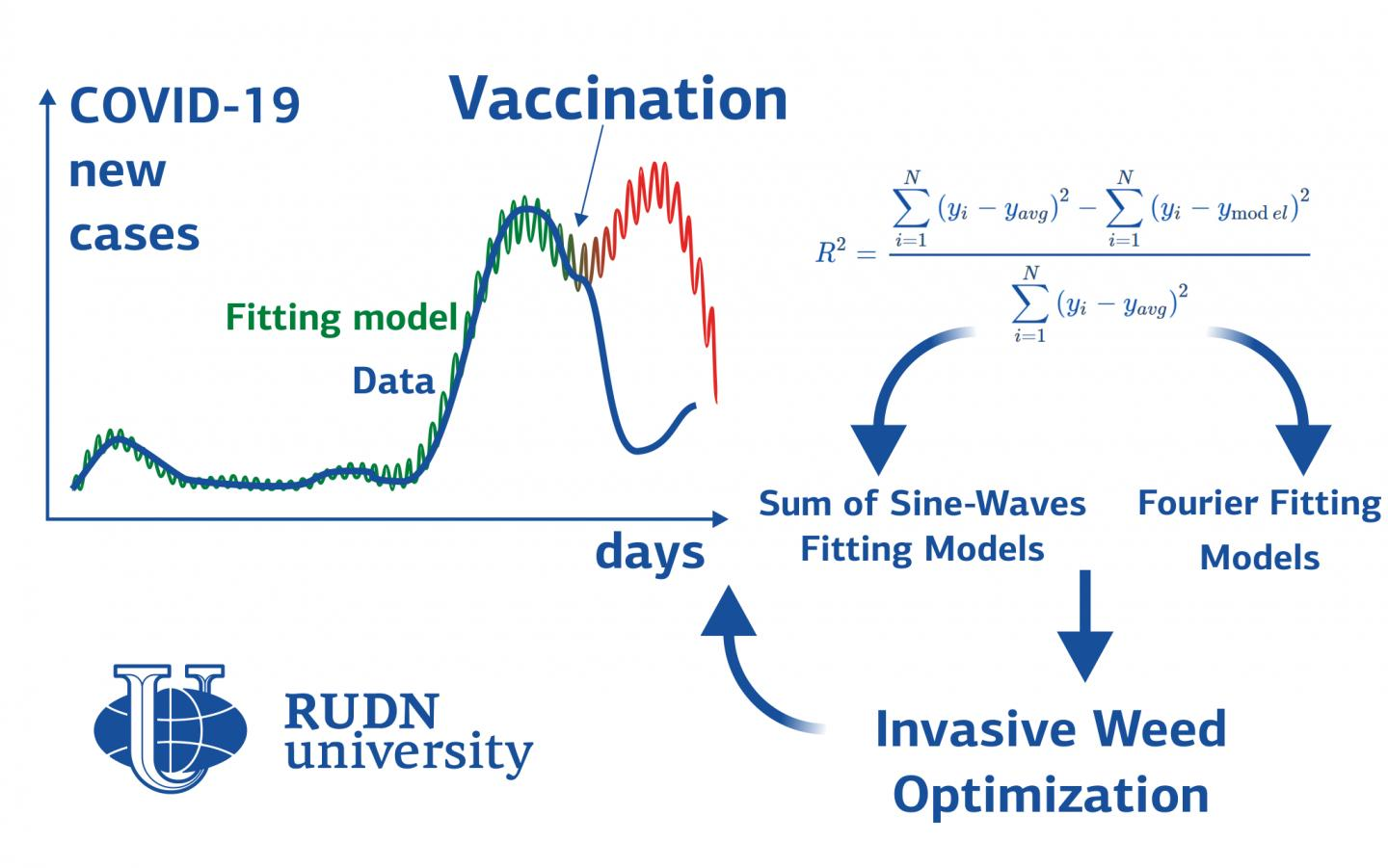News Release

# RUDN mathematician builds a COVID-19 spread model -- it shows how vaccination affects pandemic

Peer-Reviewed Publication

RUDN Universityimage: RUDN University mathematicians built a model of COVID-19 spreading based on two regression models. The mathematicians divided the countries into three groups, depending on the spreading rate and on the climatic conditions, and found a suitable mathematical approximation for each of them. Based on the model, the mathematicians predicted the subsequent waves. The forecast was accurate in countries where mass vaccination was not introduced. view more  Credit: RUDN University

RUDN University mathematicians built a model of COVID-19 spreading based on two regression models. The mathematicians divided the countries into three groups, depending on the spreading rate and on the climatic conditions, and found a suitable mathematical approximation for each of them. Based on the model, the mathematicians predicted the subsequent waves. The forecast was accurate in countries where mass vaccination was not introduced. The results are published in Mathematics.

The epidemy spreading rate within the country depends, among other things, on the climatic conditions: temperature, humidity, winds. For example, in the cold season, dry air dries the nasal mucus out which acts as a first line of defence to the virus. Therefore, a person becomes infected faster. High temperature, on the contrary, prevents the virus from surviving. Based on these considerations, professor Maria Alessandra Ragusa of RUDN University together with her colleagues from Egypt and Italy built models of the COVID-19 spread separately for three groups of countries with different climatic conditions. It turned out that the model accurately predicts the further course of the epidemic, but only until the effect of vaccination begins to affect.

"The main challenge during studying epidemics is how to predict the disease behaviour, how many people will be infected in the future, determining the pandemic peak, second wave of the disease time of action, and the total deaths after the pandemic ends. We used a new state-of-art of regression models to model daily confirmed cases and to predict the upcoming coronavirus waves in different countries.", Maria Alessandra Ragusa, professor of RUDN University.

Mathematicians have identified three groups of countries. The first category includes countries where the first wave of the pandemic lasted about 180 days. These are the countries with the lowest spreading rate, with an average annual temperature of 15-38 ? (for example, Saudi Arabia, Egypt). In the second group of countries (for example, the United Kingdom, Germany, Italy) with an average annual temperature of 2-31 ° C, the first wave lasted 90 days. Countries in this group are characterized by an average infection rate and stopping periods with low virus spreading rate. The third group includes countries with the highest spreading rate and no stopping periods, with an average annual temperature of 2-18 degrees Celsius -- for example, the United States and Russia.

For modelling, scientists used WHO data on the number of cases from March 1 to November 15, 2020. RUDN mathematicians chose the most suitable regression models -- methods for statistical research of the influence of several variables on one value. The Fourier series and the sum of the sine-waves were the most accurate for the modelling COVID-19 cases. This means that the curve of new cases of the disease is represented either as a sum of Fourier functions (they can be represented as waves of a certain frequency and amplitude), or as a sum of ordinary sine-waves.

As a result, professor Ragusa obtained the calculated values of the peak of the second or third wave in the studied countries. Different models gave close forecasts with a difference of several days. The obtained predictions were compared with the data available at that time. It turned out that the model provides fairly accurate predictions if the country does not introduce wide vaccination. For example, the calculated value of the peak of new cases in Egypt is 1481 people on January 11, 2021; the real peak occurred on December 31 with 1418 cases. In other countries, the model provides an accurate prediction until the beginning of 2021. After that, the vaccination effect takes place and the calculated values differ from the reality. For example, for Germany, the predicted and real values are close until about January 15, 2021, and on February 15 they differ by about 2.5 times.

"In our future work, we will make developments on the current predictive models considering how vaccination affects the virus spread rate", Maria Alessandra Ragusa, professor of RUDN University.

###

Disclaimer: AAAS and EurekAlert! are not responsible for the accuracy of news releases posted to EurekAlert! by contributing institutions or for the use of any information through the EurekAlert system.